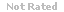Loading... Please wait...

A confidence interval is generally created when statistical tests fail to reject the null hypothesis – that is, when results are not statistically significant.

Price:
\$8.99

Product Description

1. A confidence interval is generally created when statistical tests fail to reject the null hypothesis – that is, when results are not statistically significant.
2. Chi-square tests rarely have type I errors
3. Chi-square tests are more likely to have type II (falsely rejecting the null hypothesis) errors than parametric tests.
4. For a one sample confidence interval, the interval is calculated around the calculated sample mean (m).
5. Compared to the ANOVA test, Chi-Square procedures are not powerful (able to detect small differences).
6. While rejecting the null hypothesis for the goodness of fit test means distributions differ, rejecting the null for the test of independence means the variables interact.
7. The distribution for the goodness of fit test equals k-1, where k equals the number of categories.
8. The Chi-square test is very sensitive to small differences in frequency differences.
9. The goodness of fit test can be used for a single or multiple set (rows) of data, such as comparing male and female age distributions with an expected distribution at the same time.
10. The probability that the actual population mean will be outside of a 98% confidence interval is

Customers Who Viewed This Product Also Viewed

• \$12.99
• \$22.99
• \$8.99
• \$17.99
• \$8.99

• \$8.99